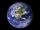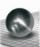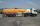Sphere A2V

Surface of the sphere is 241 mm2. What is its volume?

Result

V =  351.803 mm3

Solution:Leave us a comment of example and its solution (i.e. if it is still somewhat unclear...):Be the first to comment!Next similar examples:

1. BallsThree metal balls with volumes V1=71 cm3 V2=78 cm3 and V3=64 cm3 melted into one ball. Determine it's surface area.
2. Fit ballWhat is the size of the surface of Gymball (FIT - ball) with a diameter of 65 cm?
3. Earth's surfaceThe greater part of the earth's surface (r = 6371 km) is covered by oceans; their area is approximately 71% of the Earth's surface. What is the approximate area of the land?The surface of the sphere is 60 cm square. Calculate its radius; result round to tenth of cm.
5. Hollow sphereThe volume of the hollow ball is 3432 cm3. What is its internal radius when the wall thickness is 3 cm?
6. Sphere fallHow many percent fall volume of sphere if diameter fall 10×?
7. Sphere growthHow many times grow volume of sphere if diameter rises 10×?
8. Volume of ballFind the volume of a volleyball that has a radius of 4 1/2 decimeters. Use 22/7 for π
9. Gasoline tank cylindricalWhat is the inner diameter of the tank, which is 8 m long and contains 40 cubic cubic meters of gasoline?
10. Fifth of the numberThe fifth of the number is by 24 less than that number. What is the number?
11. Percentages52 is what percent of 93?
12. Holidays - on poolChildren's tickets to the swimming pool stands x € for an adult is € 2 more expensive. There was m children in the swimming pool and adults three times less. How many euros make treasurer for pool entry?
13. Three brothersThe three brothers have a total of 42 years. Jan is five years younger than Peter and Peter is 2 years younger than Michael. How many years has each of them?
14. Simplify 2Simplify expression: 5ab-7+3ba-9
15. Equations - simpleSolve system of linear equations: x-2y=6 3x+2y=4
16. MushroomsEva and Jane collected 114 mushrooms together. Eve found twice as much as Jane. How many mushrooms found each of them?
17. TreesAlong the road were planted 250 trees of two types. Cherry for 60 CZK apiece and apple 50 CZK apiece. The entire plantation cost 12,800 CZK. How many was cherries and apples?